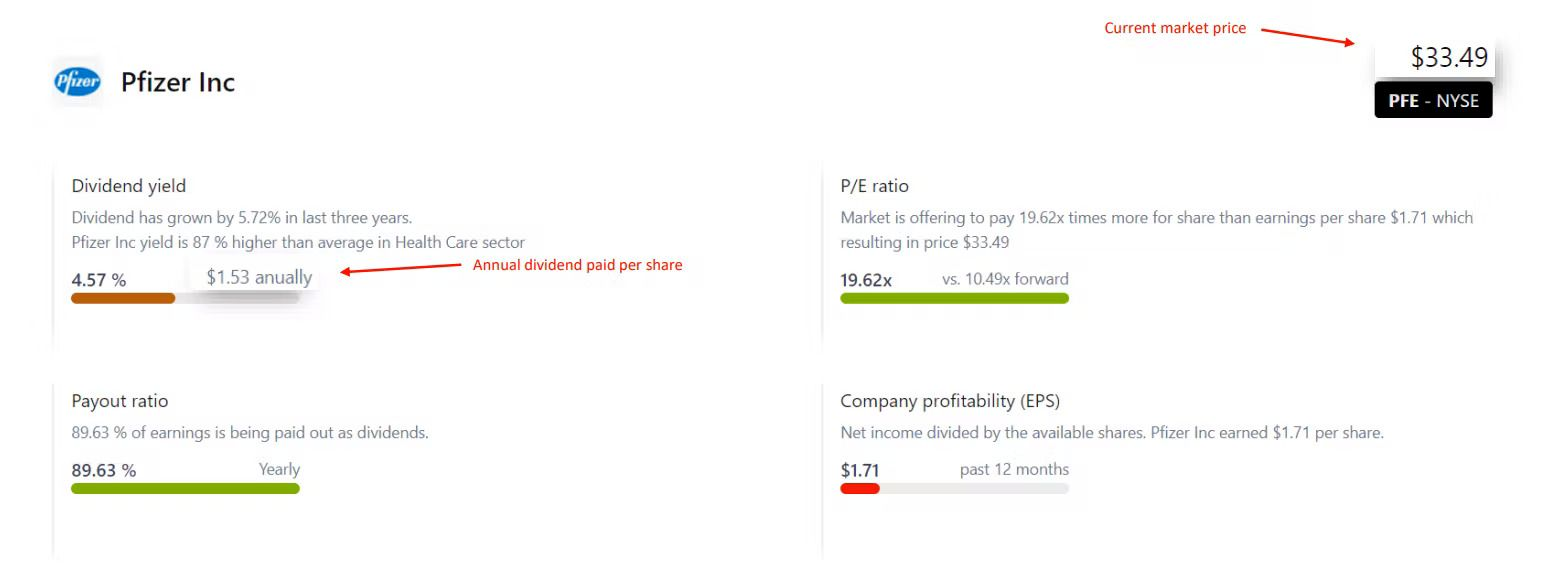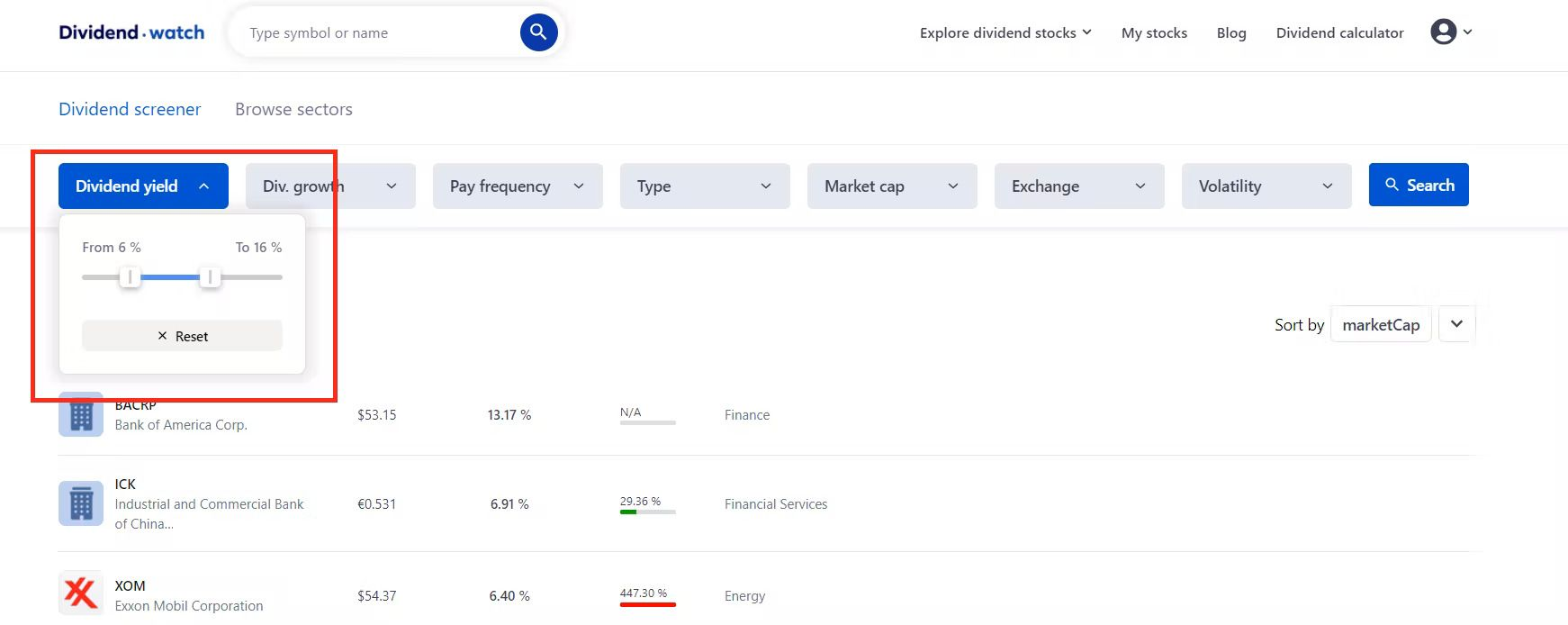# How to calculate dividend yield

Published on
03/04/2021

Dividend income is one of the best ways to earn a stable passive income and manage your time value of money. A company that rewards its shareholders with a regular and consistent dividend is considered highly attractive & is a great instrument to invest in. However, one of the most frequently asked questions by investors like you is how to analyze and pick the stocks which will offer the best returns. One of the most sought-after approaches chosen by seasoned investors is calculating dividend yield and then comparing all the individual results to pick the most rewarding stocks. In this article, we aim to provide a detailed overview of dividend yield and how you can calculate and utilize it to get the most from your investments.

## 1. Understanding Dividend Yield

Dividend yield basically shows the relationship between a particular stock’s annually paid dividend with its current market price. The most important aspect to take into consideration is that the dividend yield is the result of comparison with the current market price of the stock and hence can change drastically if the stock price fluctuates or is highly volatile. Moreover, the dividend yield is not limited to analyze the potential dividends you can earn from stocks but is a great tool to see the dividend earning potential among numerous asset classes like real estate, commodities, REITs, and many more. Therefore whether you are a beginner investor or a hedge fund manager, the dividend yield is an intrinsic part of the investment process and hence becomes an essential metric to understand thoroughly.

## 2. Calculating Dividend Yield

Even though this might seem a bit complicated to many beginner investors, calculating dividend yield is not rocket science. In order to find it, you would require essential matrices i.e. annual dividend paid per share and the share’s current market price. Once you have the data handy, remember the calculation includes only the dollar value and not percentage or any other metric, therefore you might need to convert the annual dividend paid in dollar value if it's available in percentage terms.

For example, let's look at the ongoing topic about vaccines and this Pfizer, Inc. company. To this day the annual dividend paid per share is \$1,53 and the current market price is \$33,49. Well if you divide these numbers in order of the formula below, you get 4,56%.Dividend Yield = (annual dividend paid per share / current market price) * 100

As it can be seen the formula is easy to understand and calculate, therefore can be a highly useful tool for almost every beginner investor. During the comparison among individual stocks, it should be understood that the higher the dividend yield, the better would be stock however as mentioned above it's essential to choose a stock that has low to medium volatility. This will ensure your dividend income remains constant without much risk. Conclusively, when planning your investments it is essential for you to at least take control of your basic investments. As it can be observed above understanding the concept of Dividend Yield is not as difficult as one might assume and can give many crucial insights into the stock’s performance and dividend payment history.

## 3. Find perfect stocks

We have updated our dividend screener, so you can now easily find companies that fit your investing strategy based on the Dividend Yield parameter.Report issue